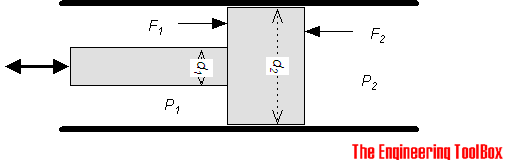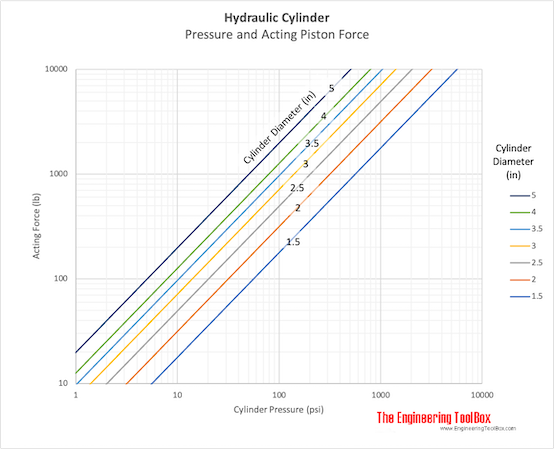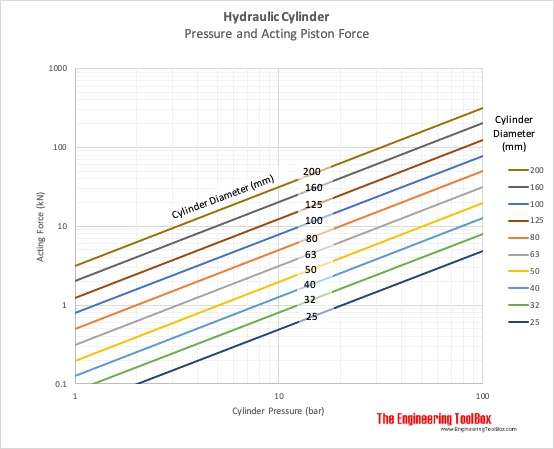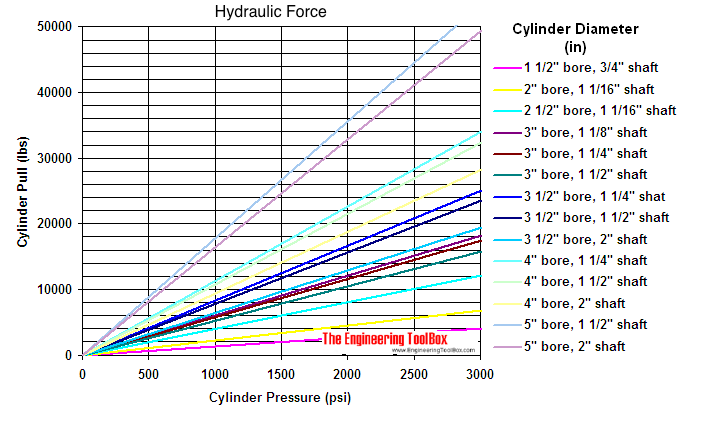Engineering ToolBox - Resources, Tools and Basic Information for Engineering and Design of Technical Applications!

# Hydraulic Force vs. Pressure and Cylinder Size

## Calculate hydraulic cylinder force.The force produced on the rod side (1) of a double acting hydraulic piston - can be expressed as

F1 = P1 (d22 - d12) / 4)                                    (1)

where

F1 = rod force (lb, N)

d1 = rod diameter (in, mm)

d2 = piston diameter (in, mm)

P1 = pressure in the cylinder on the rod side (psi, N/mm2)

• 1 bar = 105 N/m2 = 0.1 Nm/mm2

The force produced on the opposite of rod side (2) - can be expressed as

F2 = P2 (π d22 / 4)                                   (2)

where

F2 = rod force (lb, N)

P2 = pressure in the cylinder (opposite rod) (psi, N/mm2)

### Hydraulic Force Calculator

#### Imperial Units

##### Pressure acting on rod side

Piston diameter - d2 (in)

Rod diameter - d1 (in)

Cylinder pressure - P1 (psi)

##### Pressure acting on opposite of rod side

Piston diameter - d2 (in)

Cylinder pressure - d2 (psi)

#### Metric Units

##### Pressure acting on rod side

Piston diameter - d2 (mm)

Rod diameter - d1 (mm)

Cylinder pressure - P1 (bar)

##### Pressure acting on opposite of rod side

Piston diameter - d2 (mm)

Cylinder pressure - d2 (bar)

### Related Mobile Apps from The Engineering ToolBox

- free apps for offline use on mobile devices.

### Rod Force F2 diagram

- when pressure act on opposite side of rod.

#### Imperial Units###• 1 psi (lb/in2) = 144 psf (lbf/ft2) = 6,894.8 Pa (N/m2) = 6.895x10-3 N/mm2 = 6.895x10-2 bar
• 1 N/m2 = 1 Pa = 1.4504x10-4 lb/in2 = 1x10-5 bar = 4.03x10-3 in water = 0.336x10-3 ft water = 0.1024 mm water = 0.295x10-3 in mercury = 7.55x10-3 mm mercury = 0.1024 kg/m2 = 0.993x10-5 atm
• 1 lbf (Pound force) = 4.44822 N = 0.4536 kp
• 1 N (Newton) = 0.1020 kp = 7.233 pdl = 7.233/32.174 lbf = 0.2248 lbf = 1 (kg m)/s2 = 105 dyne = 1/9.80665 kgf
• 1 in (inch) = 25.4 mm
• 1 m (meter) = 39.37 in = 100 cm = 1000 mm

### Rod Force F1 diagram

- when pressure act on the same side of the rod.

#### Imperial Units## Engineering ToolBox - SketchUp Extension - Online 3D modeling!

Add standard and customized parametric components - like flange beams, lumbers, piping, stairs and more - to your Sketchup model with the Engineering ToolBox - SketchUp Extension - enabled for use with the amazing, fun and free SketchUp Make and SketchUp Pro .Add the Engineering ToolBox extension to your SketchUp from the SketchUp Pro Sketchup Extension Warehouse!

Translate

## Privacy

We don't collect information from our users. Only emails and answers are saved in our archive. Cookies are only used in the browser to improve user experience.

Some of our calculators and applications let you save application data to your local computer. These applications will - due to browser restrictions - send data between your browser and our server. We don't save this data.

## Citation

• Engineering ToolBox, (2009). Hydraulic Force vs. Pressure and Cylinder Size. [online] Available at: https://www.engineeringtoolbox.com/hydraulic-force-calculator-d_1369.html [Accessed Day Mo. Year].

Modify access date.

. .

#### Scientific Online Calculator6 24